# Top 5 Methods of Capital Budgeting | Managerial Economics

The following points highlight the top five methods of capital budgeting.

The methods are: 1. Degree of Urgency Method 2. First year’s Performance Method 3. Pay Back Period Method 4. Rate of Return Method 5. Present Value Method.

#### Capital Budgeting Method # 1. Degree of Urgency Method:

The project work which is most urgent i.e., which cannot be postponed is taken first.

For example—If there is a break-down in the production process due to loss of any parts of the machinery which requires immediate replacement in order to avoid disruption in production.

It shall be given first priority over all their projects pending consideration with the management without any delay. This capital budgeting method is very simple. The urgent project or work may be undertaken first. But this is not a scientific method for evaluating the economic worth of the project.

Defects:

The important defects of this method are as follows:

(a) This is not a Methodical Analysis:

The action taken may be correct in most cases which have been considered as coincidence. Urgency cannot be a convincing influence in case where projected outlay is large and far reaching in effect.

(b) Here, there is Role of Persuasion:

In this each department in charge persuades the top management to assign priority for his department project. In this decisions are taken not on economic consideration but on the basis of ‘Power of persuasion’ of the individual concerned.

#### Capital Budgeting Method # 2. First Year’s Performance Method:

In this the investment projects are evaluated on the basis of their impact on evenness and expenses in the first year. If the increased revenue from added sales or equipment exceeds the expenses resulting from the improved techniques or equipment, the investment is accepted, otherwise it is rejected.

This capital budgeting method is simple though not popular. It takes into consideration only the first year’s results and ignores subsequent revenues and the value of money.

#### Capital Budgeting Method # 3. Pay Back Method:

This is also known as payoff, pay out or replacement period method. This is widely recognised as traditional method of evaluating capital projects. It is based on the principle that every capital expenditure pays itself back over a number of years, it attempts to measure the period of time. Further, the pay-back period is that period where the total earnings or net cash inflow from investment equals the total outlays.

Taking into account a number of considerations standard recoupment period is fixed by the management. This period of recoupment of investment known as the pay-back period is fixed by a Rule of Thumb. This period varies from 3 to 5 years.

This method is useful in evaluation of projects with high uncertainty caused by political instability, rapid technological changes and quick limitation possibilities etc. This makes it clear that no profit arises till the pay-back period is over:

Formula:

Pay Back Period = Original Investment/Annual Cash Flow

The calculation on pay-back period takes a cumulative form if the annual cash inflow is uneven. The annual cash inflow is accumulated till the recovery of investment. An asset or capital expenditure that pays back early is comparatively preferred.

#### Capital Budgeting Method # 4. Average Rate of Return Method:

This method is also known as:

(i) Accounting rate of return method, or

(ii) Financial statement method, or/as

(iii) Un-adjusted rate of return method.

In this capital projects is prepared in order of earnings, selecting projects which yield the highest earnings and rejecting others.

This can be explained in the following ways:

(i) Average rate of return,

(ii) Earnings per unit of money invested,

(iii) Return on average amount of investment.

(i) Average Rate of Return:

Under this method all the earnings after depreciation are added and divided by the project’s economic life. After this figure of average earnings over the period is obtained it is divided by average investment over the period which is the simple arithmetic mean of the values of assets of the beginning and end of the useful life of the asset which is always zero at the latter point of time.

While adopting this method it should be kept in mind that average investment in a project is always one half of the original investment.

Accepted Formulae is: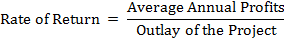Thus, the average rate of return method considers whole earnings over the entire life of the asset. A project which shows higher percentage of return will be acceptable.

(ii) Earnings per Unit of Money Invested:

In this, the total net earnings are divided by the total investment to arrive at the average rate of return per unit of amount invested in the project as per the formulae written under: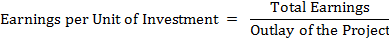This project deserves to be selected which has higher earnings per unit.

(ii) Return on Average Amount of Investment:

The percentage return on average amount of investment is calculated as per the following formulae: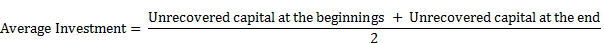Or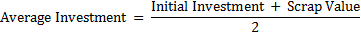When the equipment has no scrap value.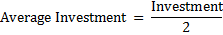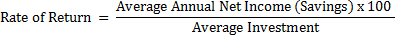Here, Average Annual Net Income = Average Annual Cash Inflow – (minus) Depreciation.

#### Capital Budgeting Method # 5. Present Value Method:

This method is also known as:

(a) The adjusted rate of return, or

(b) Internal rate of return method, or

(c) Required earning ratio.

This method has been recognised as the most meaningful techniques for financial decisions regarding future commitments and projects. It is based on the assumption that future rupee value cannot be returned or cash inflows with the amount of investment or cash outflows, both must be stated on a present value basis if the time value of money is to be given due importance.

There are four types of Present Value method:

(a) Net Present Value Method

(b) Internal Rate of Return Method

(c) Profitability Index Method

(d) Terminal Value Method.

(a) Net Present Value Method:

This is also called as Net Gain Method or Excess Present Value Method. It takes into account all income whenever received. A required rate of return is assumed and a comparison is made between the present value of cash flows at different times and original investment order to determine the prospective profitability.

It is based on the principle that if the present value of cash inflow discounted at a specified rate return equals or exceeds the amount of investment required the investment proposal should be accepted.

(b) Internal Rate of Return Method:

This is also known as:

(i) Time Adjusted Rate of Return method

(ii) Yield Rate Method,

(iii) Investors Method,

(iv) Marginal Efficiency of Capital Method.

According to the National Association of Accounts U.S.A.; Time Adjusted Rate of Return is the maximum rate of interest that could be paid for the capital employed over the life of an investment without loss of the profit. In this method rate of interest or discount is calculated.

Internal rate of return is the rate of interest or discount at which the present value of expected cash flows is equal to the total investment outlay. This rate is usually found by trial and error method. For example—Select an arbitrary rate of interest and find the present value of cash flows during the life of investment at the selected rate.

(c) Profitability Index Method or Benefit Cash Ratio:

The present value profitability index is prepared to establish relationship between cash-inflows and the amount of investment. In this, the higher the profitability index, the more desirable is the investment. The index provides a ready compatibility indices for various projects the financial manager can rank them in order to their respective rates of profitability.

(d) Terminal Value Method:

In this method, the timing of the cash flows and outflows are separated more distinctly. It is assumed that each cash inflow is re-invested in another asset at a certain rate of return from the moment, it is received until the termination of the project.

Now, the present value of the total compounded sum is calculated and it is compared with the initial-cash outflow. This method incorporates the advantage of re-investment of cash inflows by compounding and then discounting it. It is best suited to cash budgeting requirements. Its major practical problem lies in projecting future rates of interest at which the intermediate cash-inflow will be re-invested.